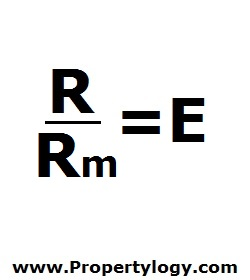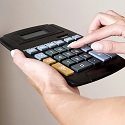Formula - Economic Rent Per Room/Unit/Square Foot | Propertylogy
Don't Miss

# Formula – Economic Rent Per Room/Unit/Square Foot

By on June 21, 2018

The first step to calculating the value of property using the income approach is to work out the economic rent.

When the economic rent is required for a multi-unit property, then the appraiser has to determine whether to base the formula on per square foot, per room, per square foot, or what he judges to be appropriate.

Economic Rent Per Square FootR/S = E

R – Rent per period

S – Square feet

E – Economic rent per square foot

Economic Rent Per RoomR/Rm = E

R – Rent per period

Rm – Number of rooms

E – Economic rent per room

Economic Rent Per UnitR/U = E

R – Rent per period

U – Number of units

E – Economic rent per unit

The economic rent is based on similar properties in the area in question and the market rental for these properties.

The role of the appraiser is critical in identifying what will be the basis of calculation in order to work out the numbers for investment analysis.

You May Also Like...##### How to burn more calories in the office

Send this to a friend